# Common Core: 7th Grade Math : Describe the Two-Dimensional Figures that Result from Slicing Three-Dimensional Figures: CCSS.Math.Content.7.G.A.3

## Example Questions

← Previous 1

### Example Question #1 : Describe The Two Dimensional Figures That Result From Slicing Three Dimensional Figures: Ccss.Math.Content.7.G.A.3

What is the two dimensional shape that is created when the shape provided is sliced vertically?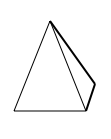Triangle

Trapezoid

Square

Rectangle

Triangle

Explanation:

A vertical cut is an up and down cut.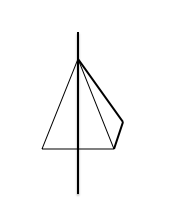This shape is a right rectangular pyramid, which has a base of a rectangle and sides in the shape of triangles; thus,  a vertical cut would make a triangle.

### Example Question #1 : Describe The Two Dimensional Figures That Result From Slicing Three Dimensional Figures: Ccss.Math.Content.7.G.A.3

What is the two dimensional shape that is created when the shape provided is sliced vertically?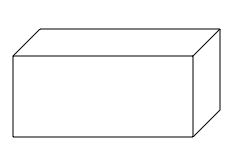Trapezoid

Triangle

Right triangle

Rectangle

Rectangle

Explanation:

A vertical cut is an up and down cut.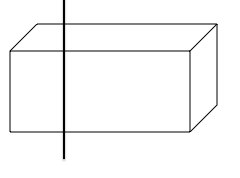This shape is rectangular prism, which has a base and sides in the shape of rectangles; thus, the two dimensional shape is a rectangle.

### Example Question #1 : Describe The Two Dimensional Figures That Result From Slicing Three Dimensional Figures: Ccss.Math.Content.7.G.A.3

What is the two dimensional shape that is created when the shape provided is sliced vertically?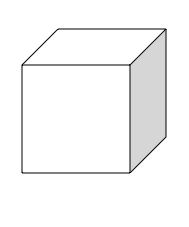Trapezoid

Triangle

Rectangle

Square

Square

Explanation:

A vertical cut is an up and down cut.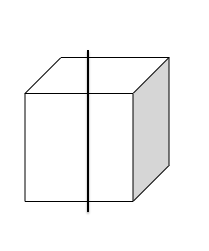This shape is cube, which has a base and sides in the shape of squares; thus, the two dimensional shape is a square.

### Example Question #2 : Describe The Two Dimensional Figures That Result From Slicing Three Dimensional Figures: Ccss.Math.Content.7.G.A.3

What is the two dimensional shape that is created when a rectangular prism is sliced vertically?

Rectangle

Trapezoid

Right triangle

Triangle

Rectangle

Explanation:

A vertical cut is an up and down cut.This shape is rectangular prism, which has a base and sides in the shape of rectangles; thus, the two dimensional shape is a rectangle.

### Example Question #1 : Describe The Two Dimensional Figures That Result From Slicing Three Dimensional Figures: Ccss.Math.Content.7.G.A.3

What is the two dimensional shape that is created when a cube is sliced vertically?

Rectangle

Square

Triangle

Right triangle

Square

Explanation:

A vertical cut is an up and down cut.This shape is cube, which has a base and sides in the shape of squares; thus, the two dimensional shape is a square.

### Example Question #3 : Describe The Two Dimensional Figures That Result From Slicing Three Dimensional Figures: Ccss.Math.Content.7.G.A.3

What is the two dimensional shape that is created when a right rectangular pyramid is sliced vertically?

Square

Trapezoid

Rectangle

Triangle

Triangle

Explanation:

A vertical cut is an up and down cut.This shape is a right rectangular pyramid, which has a base of a rectangle and sides in the shape of triangles; thus,  a vertical cut would make a triangle.

### Example Question #1 : Describe The Two Dimensional Figures That Result From Slicing Three Dimensional Figures: Ccss.Math.Content.7.G.A.3

What is the two dimensional shape that is created when the shape provided is sliced horizontally?Triangle

Rectangle

Right Triangle

Cube

Rectangle

Explanation:

A horizontal cut is a side to side cut.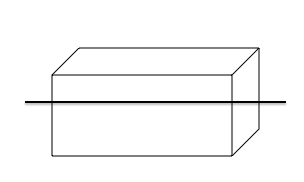This shape is rectangular prism, which has a base and sides in the shape of rectangles; thus, the two dimensional shape is a rectangle.

### Example Question #1 : Describe The Two Dimensional Figures That Result From Slicing Three Dimensional Figures: Ccss.Math.Content.7.G.A.3

What is the two dimensional shape that is created when the shape provided is sliced horizontally?Rectangle

Square

Triangle

Trapezoid

Square

Explanation:

A horizontal cut is a side to side cut.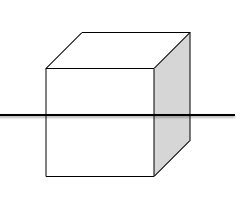This shape is cube, which has a base and sides in the shape of squares; thus, the two dimensional shape is a square.

### Example Question #9 : Describe The Two Dimensional Figures That Result From Slicing Three Dimensional Figures: Ccss.Math.Content.7.G.A.3

What is the two dimensional shape that is created when the shape provided is sliced horizontally?Right triangle

Trapezoid

Triangle

Rectangle

Rectangle

Explanation:

A horizontal cut is a side to side cut.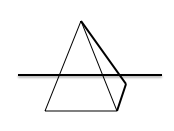This shape is a right rectangular pyramid, which has a base of a rectangle and sides in the shape of triangles; thus, a horizontal cut would make a rectangle.

### Example Question #1 : Describe The Two Dimensional Figures That Result From Slicing Three Dimensional Figures: Ccss.Math.Content.7.G.A.3

What is the two dimensional shape that is created when a rectangular prism is sliced horizontally?

Trapezoid

Rectangle

Triangle

Right triangle

Rectangle

Explanation:

A horizontal cut is a side to side cut.This shape is rectangular prism, which has a base and sides in the shape of rectangles; thus, the two dimensional shape is a rectangle.

← Previous 1

### All Common Core: 7th Grade Math Resources